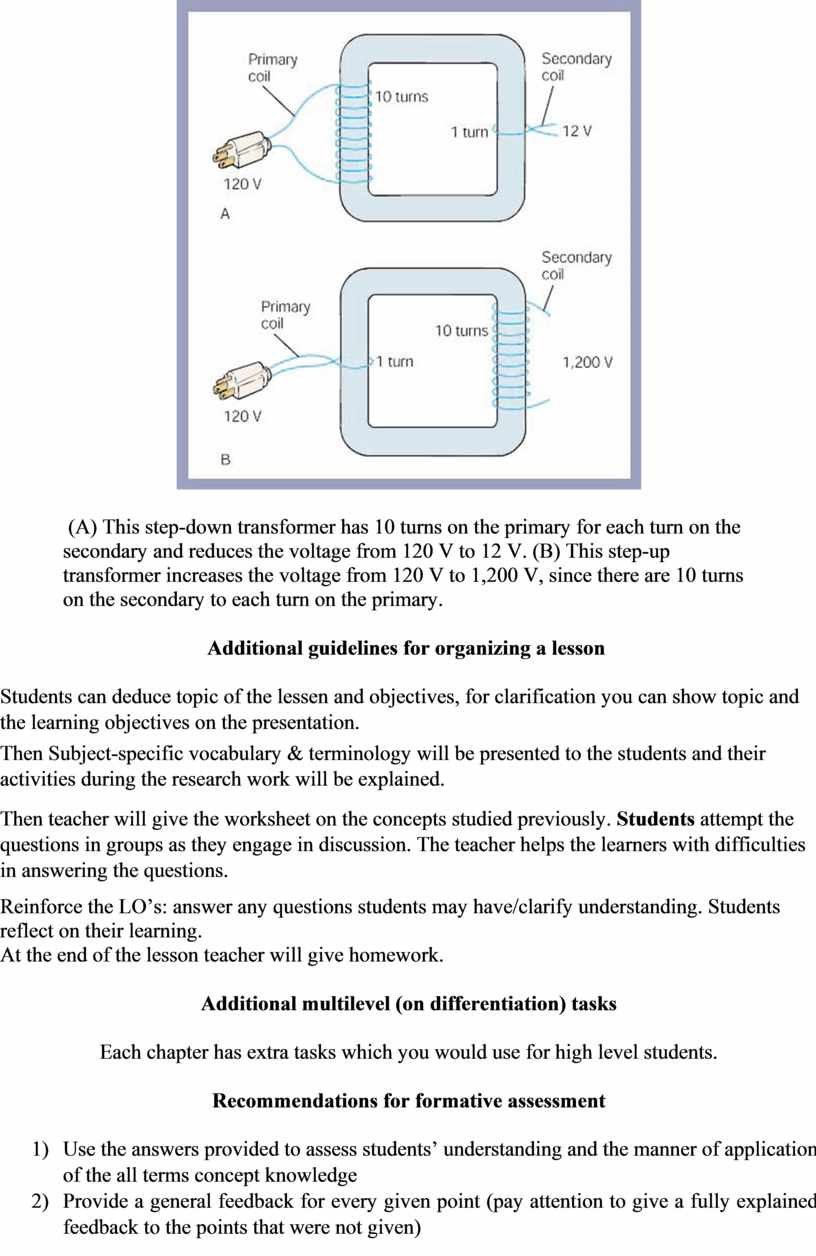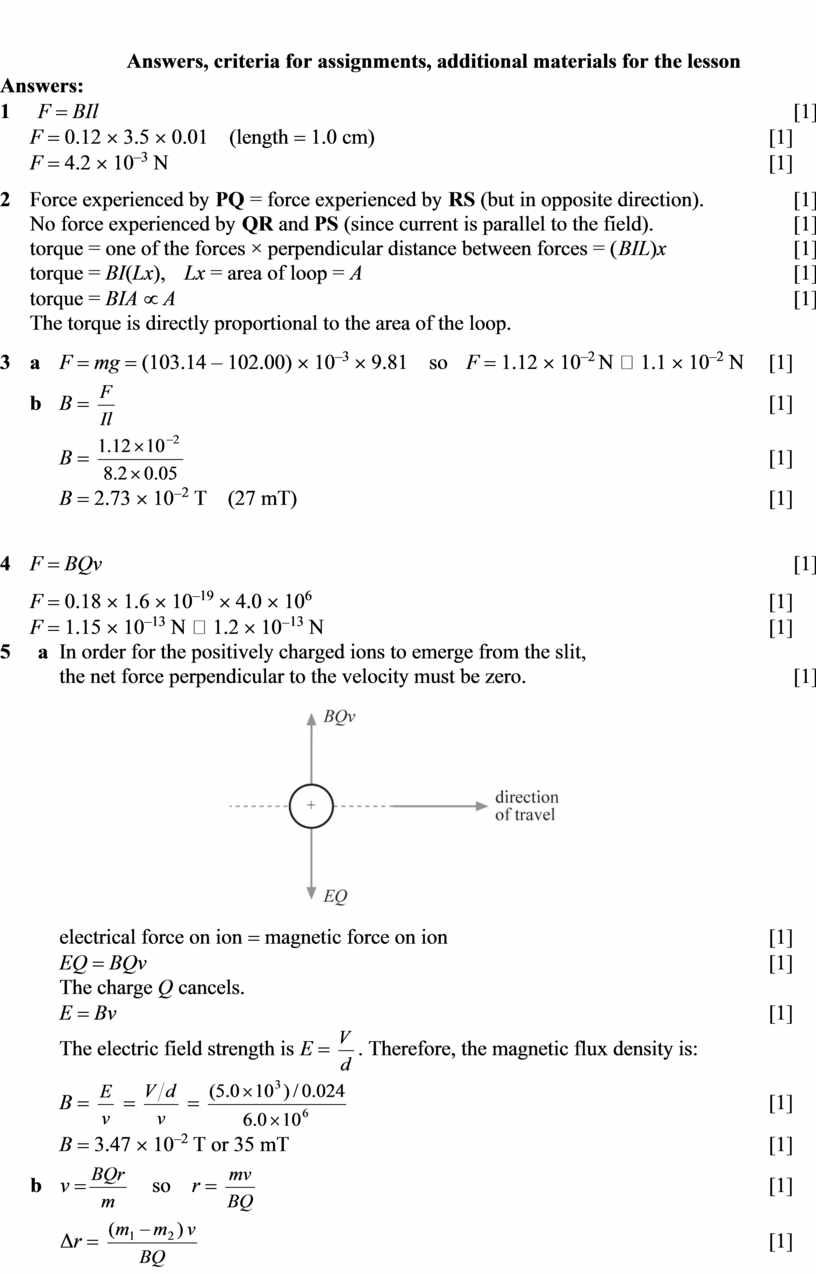PHY_10_V1_TG_Revision term 4
Оценка 4.9

# PHY_10_V1_TG_Revision term 4

Оценка 4.9
docx
07.05.2020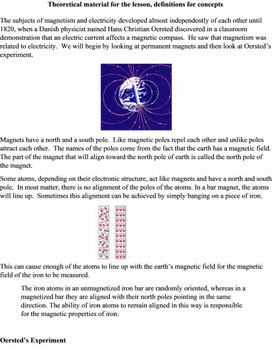PHY_10_V1_TG_Revision term 4.docx

Theoretical material for the lesson, definitions for concepts

The subjects of magnetism and electricity developed almost independently of each other until 1820, when a Danish physicist named Hans Christian Oersted discovered in a classroom demonstration that an electric current affects a magnetic compass.  He saw that magnetism was related to electricity.  We will begin by looking at permanent magnets and then look at Oersted’s experiment.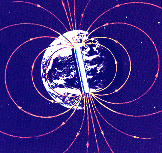Magnets have a north and a south pole.  Like magnetic poles repel each other and unlike poles attract each other.  The names of the poles come from the fact that the earth has a magnetic field.  The part of the magnet that will align toward the north pole of earth is called the north pole of the magnet.

Some atoms, depending on their electronic structure, act like magnets and have a north and south pole.  In most matter, there is no alignment of the poles of the atoms. In a bar magnet, the atoms will line up.  Sometimes this alignment can be achieved by simply banging on a piece of iron.  This can cause enough of the atoms to line up with the earth’s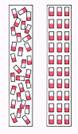magnetic field for the magnetic field of the iron to be measured.

The iron atoms in an unmagnetized iron bar are randomly oriented, whereas in a magnetized bar they are aligned with their north poles pointing in the same direction. The ability of iron atoms to remain aligned in this way is responsible for the magnetic properties of iron.

Oersted’s Experiment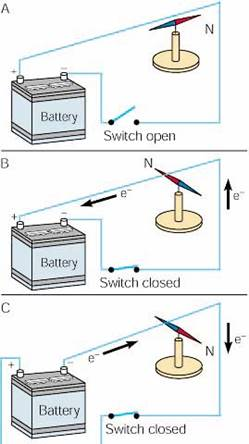Oersted discovered that a compass needle below a wire (A) pointed north when there was not a current, (B) moved at right angles when a current flowed one way, and (C) moved at right angles in the opposite direction when the current was reversed.

The Right Hand Rule:

What we can determine from this is that an electrical current produces a magnetic field .  A magnetic field surrounds the wire as shown below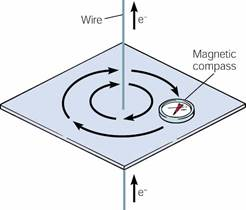A magnetic compass shows the presence and direction of the magnetic field around a straight length of current-carrying wire.

This effect can be summarized by the right hand rule.  This rule states that if you put the thumb of your right hand in the direction of the current, the magnetic field will wrap around the wire the same way your fingers will.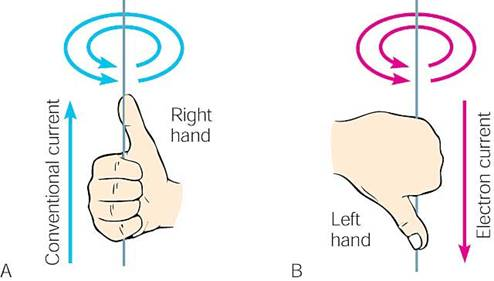Use (A) a right-hand rule of thumb to determine the direction of a magnetic field around a conventional current.  Remember that most people write current going from positive to negative.  If your stubbornness requires you to thing about electron flow, you will have to reverse everything and use the left-hand rule of thumb.

Electromagnets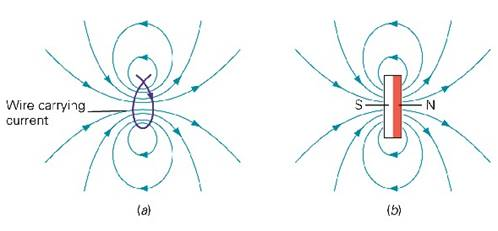Forming a wire into a loop causes the magnetic field to pass through the loop in the same direction.   Notice that all sides of the loop, by using the right hand rule, push the magnetic field the same direction.  This gives one side of the loop a north pole and the other side a south pole.  The magnetic field of the loop is the same of that as a bar magnet.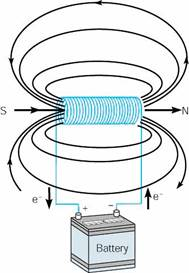When a current is run through a cylindrical coil of wire, a solenoid, it produces a magnetic field like the magnetic field of a bar magnet.

Magnetic force and current.

Because a wire carrying a current away from you, represented by the black dot, has a magnetic field it will be affected by the magnetic field of a magnet.  The yellow and red boxes represent the ends of the magnet.  The field of the magnet is in green.  The circular magnetic field of the wire is shown.  Notice how at the top the fields are lined up and repelling and at the bottom the fields are opposite and attracting.  The wire, if it can move, feels a force down called the Lorentz force.  This force is perpendicular to both the magnetic field of the magnet and the current of the wire.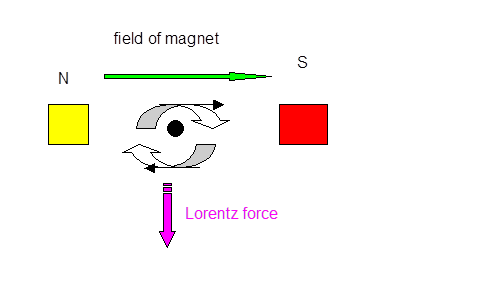Motors

http://webphysics.ph.msstate.edu/jc/library/20-3/index.html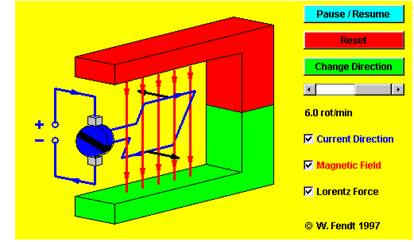An electric motor, is a machine which converts electrical energy into mechanical (rotational or kinetic) energy.  A current causes the coil to rotate mechanically.  For this to occur

In the motor a current is passed through a loop, which is immersed in a magnetic field. A force exists on the top leg of the loop, which pushes the loop left, while a force on the bottom leg of the loop pushes the loop right. The net effect of these forces is to rotate the loop in the direction indicated.  At some point, to keep the loop rotating it is necessary to switch the direction of the current. This is done on this applet when the loop is horizontal.

Induction

Electromagnetic induction refers to the production of a current in a wire when there is relative motion between the wire and a magnetic field.  This connection was discovered by Faraday, who found that changing magnetic fields though loops of wire will cause currents to be induced.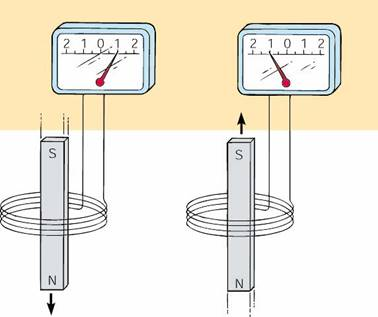In the above picture, a current is induced in a coil of wire moved through a magnetic field. The direction of the current depends on the direction of motion. These induced currents only exist as long as the magnet is moving, and will die off when the magnet becomes stationary.

It is interesting to note that the current flows so as to create a magnetic field to oppose the change created by moving the bar magnet.  This feature that the magnetic effects of the induced current are such as to oppose the external change is known as Lenz's law. The induction of currents from changing magnetic fields has a number of important applications, including, odiously, the electrical generator.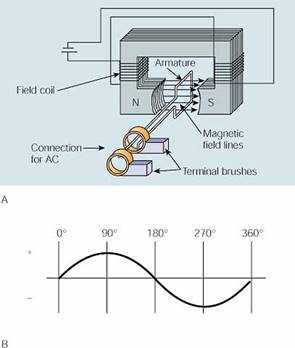(A) Schematic of a simple alternator (ac generator) with one output loop. (B) Output of the single loop turning in a constant magnetic field, which alternates the induced current each half cycle.

Transformers

A transformers useful for changing the voltage of an alternating current circuit.  In a transformer an alternating current in one coil of wire creates a changing magnetic field.  This changing magnetic field induces an alternating current in another nearby coil. Depending on the ratio of turns of the coils, the induced current can have a voltage that is larger, smaller, or the same as that of the primary current.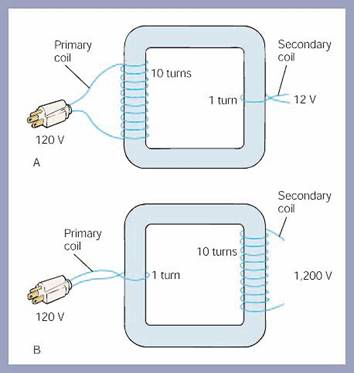(A) This step-down transformer has 10 turns on the primary for each turn on the secondary and reduces the voltage from 120 V to 12 V. (B) This step-up transformer increases the voltage from 120 V to 1,200 V, since there are 10 turns on the secondary to each turn on the primary.

Additional guidelines for organizing a lesson

Students can deduce topic of the lessen and objectives, for clarification you can show topic and the learning objectives on the presentation.

Then Subject-specific vocabulary & terminology will be presented to the students and their activities during the research work will be explained.

Then teacher will give the worksheet on the concepts studied previously. Students attempt the questions in groups as they engage in discussion. The teacher helps the learners with difficulties in answering the questions.

Reinforce the LO’s: answer any questions students may have/clarify understanding. Students reflect on their learning.

At the end of the lesson teacher will give homework.

Each chapter has extra tasks which you would use for high level students.

Recommendations for formative assessment

1)      Use the answers provided to assess students’ understanding and the manner of application of the all terms concept knowledge

2)      Provide a general feedback for every given point (pay attention to give a fully explained feedback to the points that were not given)

3)      You can change the numbers in calculations according to the student’s ability

1     F = BIl                                                                                                                                     

F = 0.12 ´ 3.5 ´ 0.01 (length = 1.0 cm)                                                                           
F
= 4.2 ´ 10–3 N                                                                                                                   

2    Force experienced by PQ = force experienced by RS (but in opposite direction).                 
No force experienced by QR and PS (since current is parallel to the field).                           
torque = one of the forces × perpendicular distance between forces = (BIL)x                        
torque = BI(Lx),
Lx = area of loop = A                                                                                  
torque = BIA
µ A                                                                                                                      
The torque is directly proportional to the area of the loop.

3    a    F = mg = (103.14 – 102.00) ´ 10–3 ´ 9.81soF = 1.12 ´ 10–2 N  1.1 ´ 10–2 N     

b   B =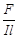B
=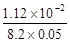B
= 2.73 ´ 10-2 T(27 mT)                                                                                          

4    F = BQv                                                                                                                                    

F = 0.18 ´ 1.6 ´ 10–19 ´ 4.0 ´ 106                                                                                                                    

F = 1.15 ´ 10–13 N 1.2 ´ 10-13 N                                                                                     

5     In order for the positively charged ions to emerge from the slit,
the net force perpendicular to the velocity must be zero.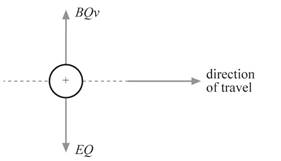electrical force on ion = magnetic force on ion                                                             

EQ = BQv                                                                                                                       

The charge Q cancels.

E = Bv                                                                                                                             

The electric field strength is E =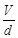. Therefore, the magnetic flux density is:

B =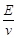=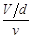=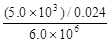B = 3.47 ´ 10–2 T or 35 mT                                                                                            

b    v =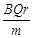so r =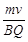Δr
=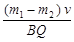6    a    distance = speed ´ time = 2.0 ´ 1.0 = 2.0 m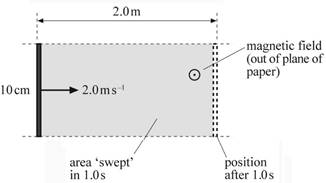b   Area swept = length ´ distance travelled = 0.10 ´ 2.0 = 0.20 m2                                  

c    Change in magnetic flux = area swept ´ magnetic flux density                                    
change in magnetic flux
= 0.20 ´ 0.050 = 1.0 ´ 10-2 Wb                                              

d   Magnitude of e.m.f. = rate of change of magnetic flux linkage                                     
E
=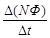(N = 1)
E
=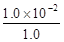= 1.0 ´ 10-2 V (1 Wb s-1 = 1 V)                                                         

e    E = Blv = 0.050 ´ 2.0 ´ 0.10 = 1.0 ´ 10-2 V                                                                  

7    Initial magnetic flux = BA = 0.060 ´ π ´ (1.2 ´ 10-2)2                                                            

initial magnetic flux = 2.72 ´ 10-5 Wb                                                                                

final magnetic flux = –2.72 ´ 10-5 Wb (since the field is reversed)                                   

average magnitude of induced e.m.f. = rate of change of magnetic flux linkage

E =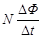, so E = 2000 ´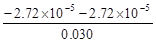E = 3.62 V or 3.6 V (magnitude only)                                                                                 

List of useful links and literature

Jancoli, Zambak and A level books

Скачано с www.znanio.ru

## Theoretical material for the lesson, definitions for concepts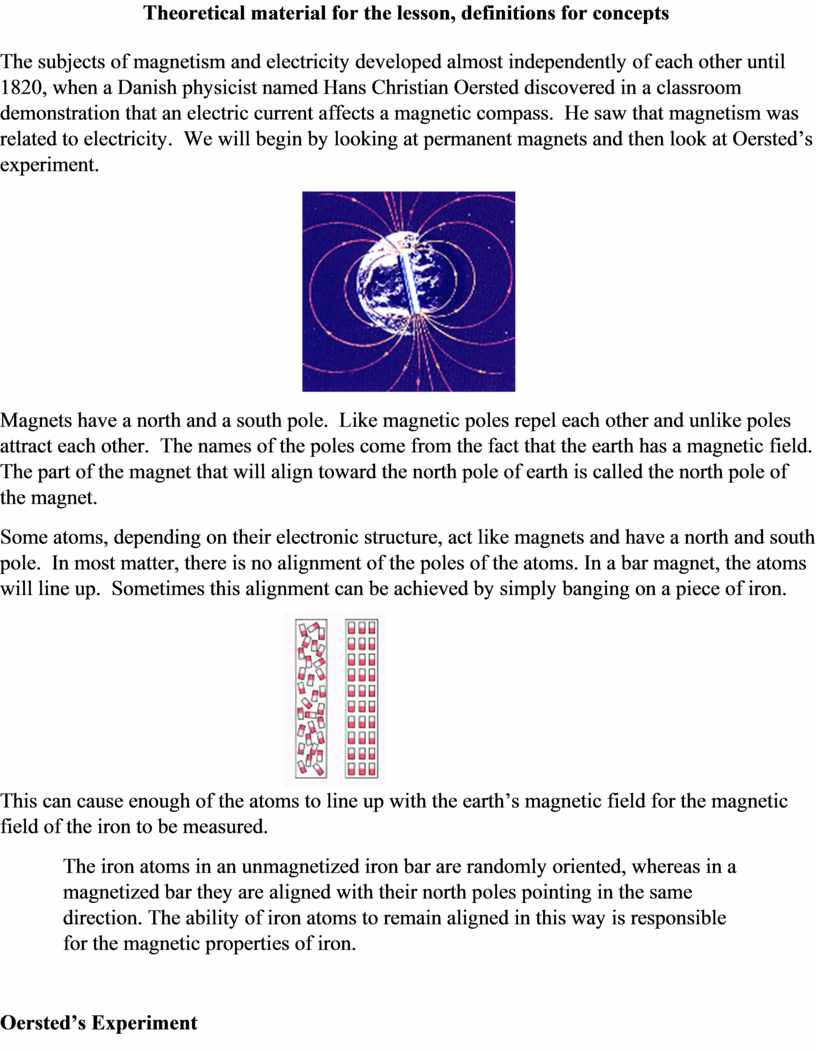## Oersted discovered that a compass needle below a wire (A) pointed north when there was not a current, (B) moved at right angles when a…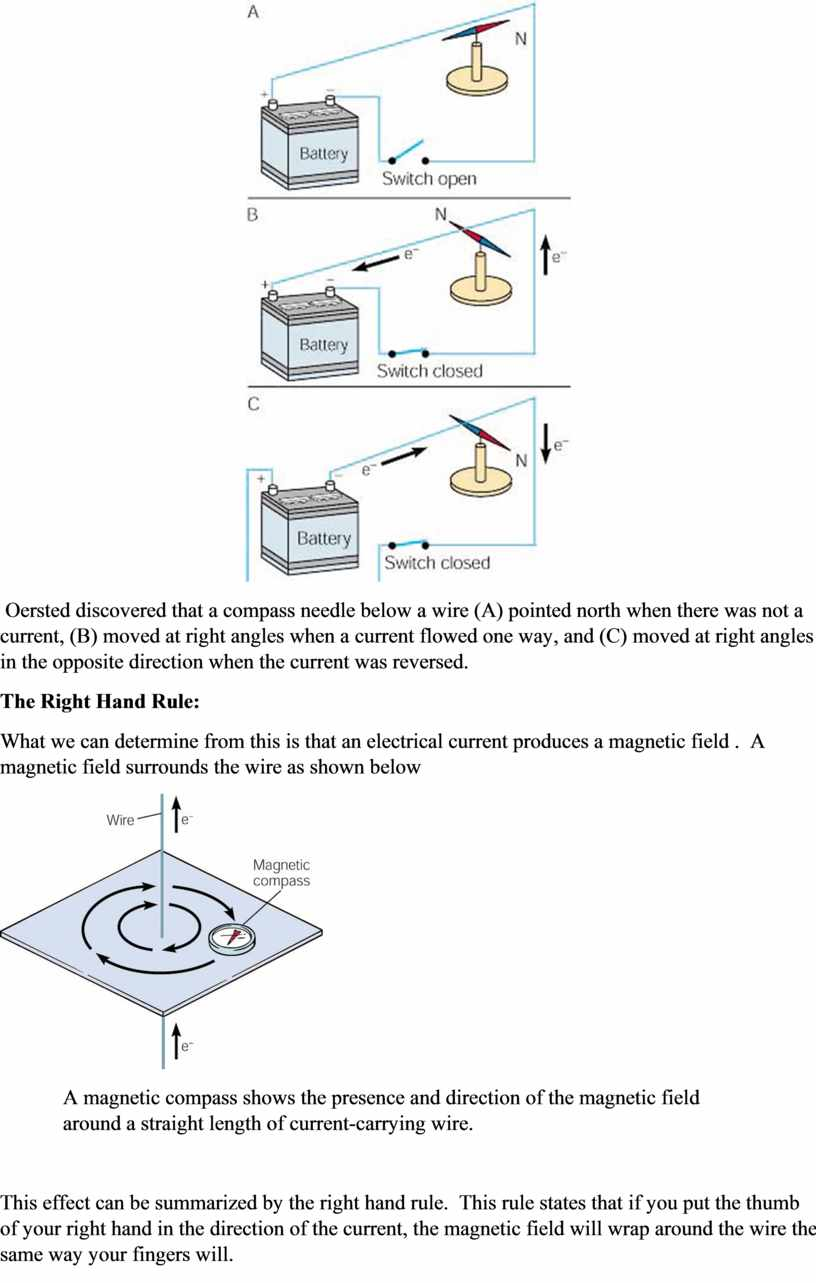## Use (A) a right-hand rule of thumb to determine the direction of a magnetic field around a conventional current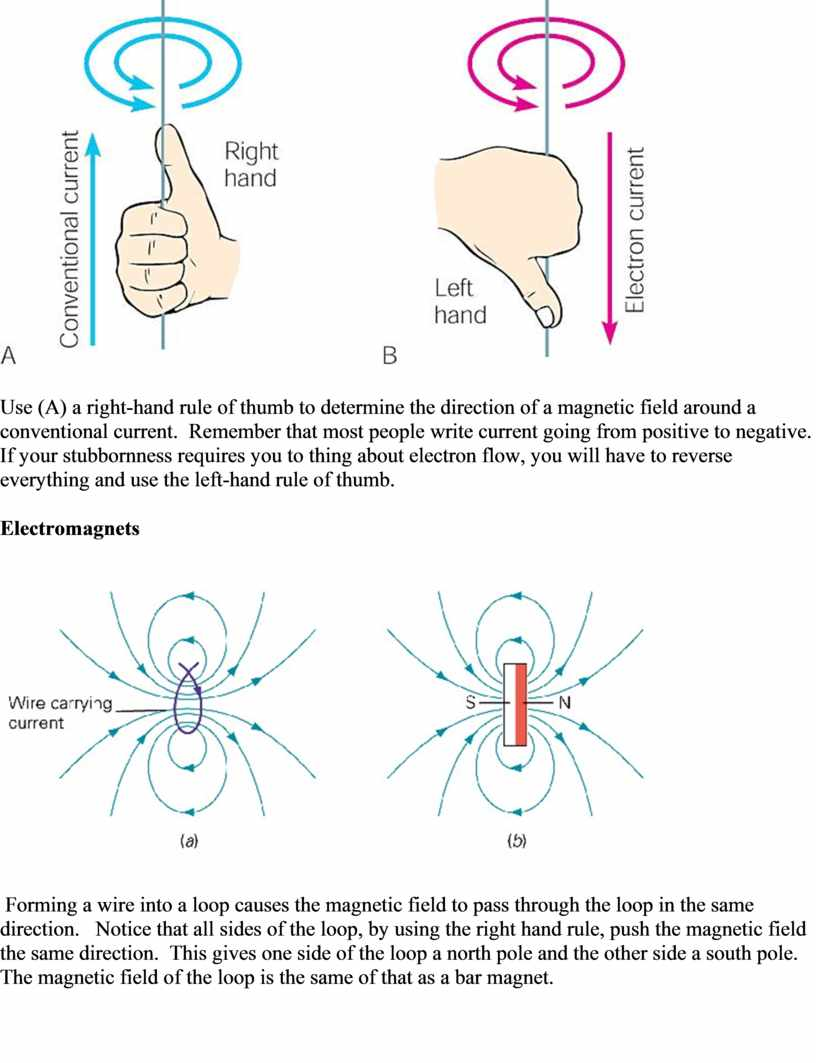## When a current is run through a cylindrical coil of wire, a solenoid, it produces a magnetic field like the magnetic field of a bar…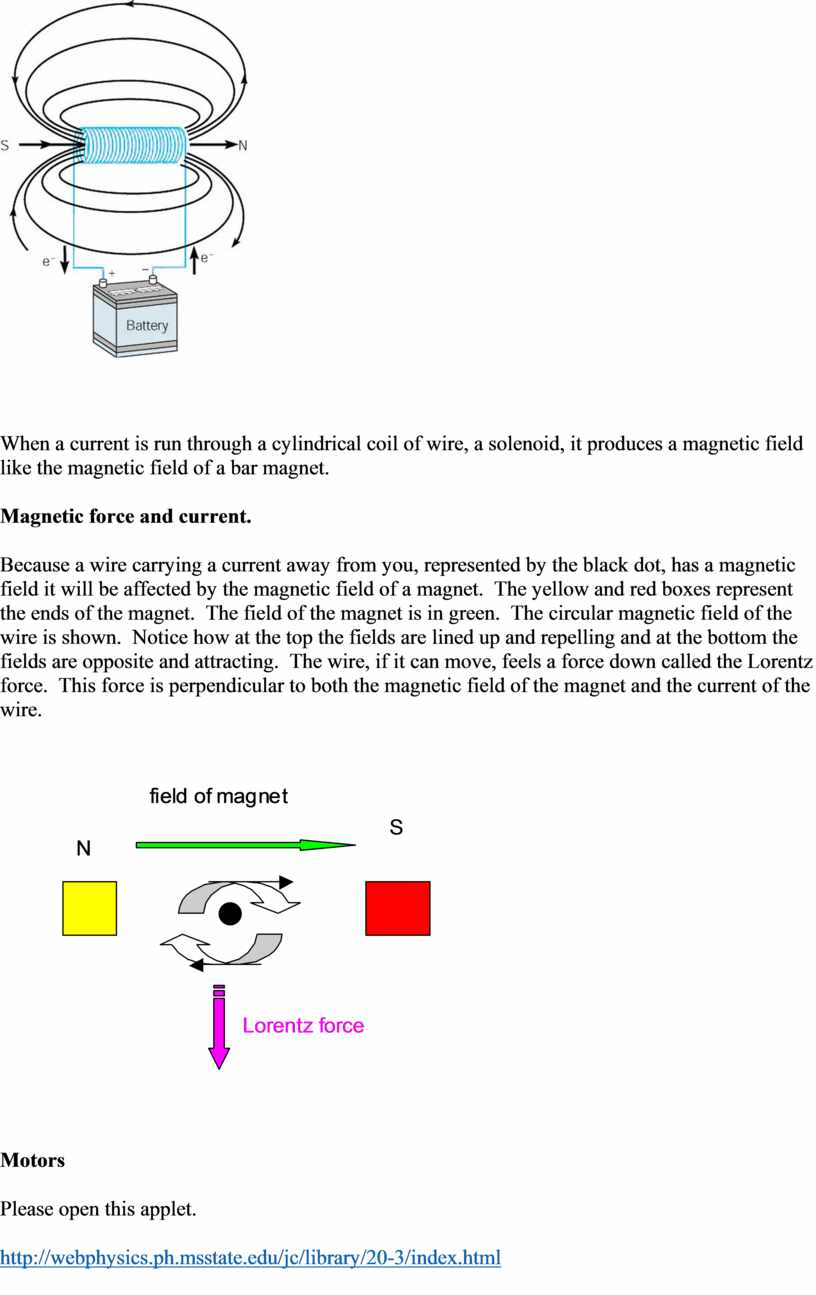## An electric motor , is a machine which converts electrical energy into mechanical (rotational or kinetic) energy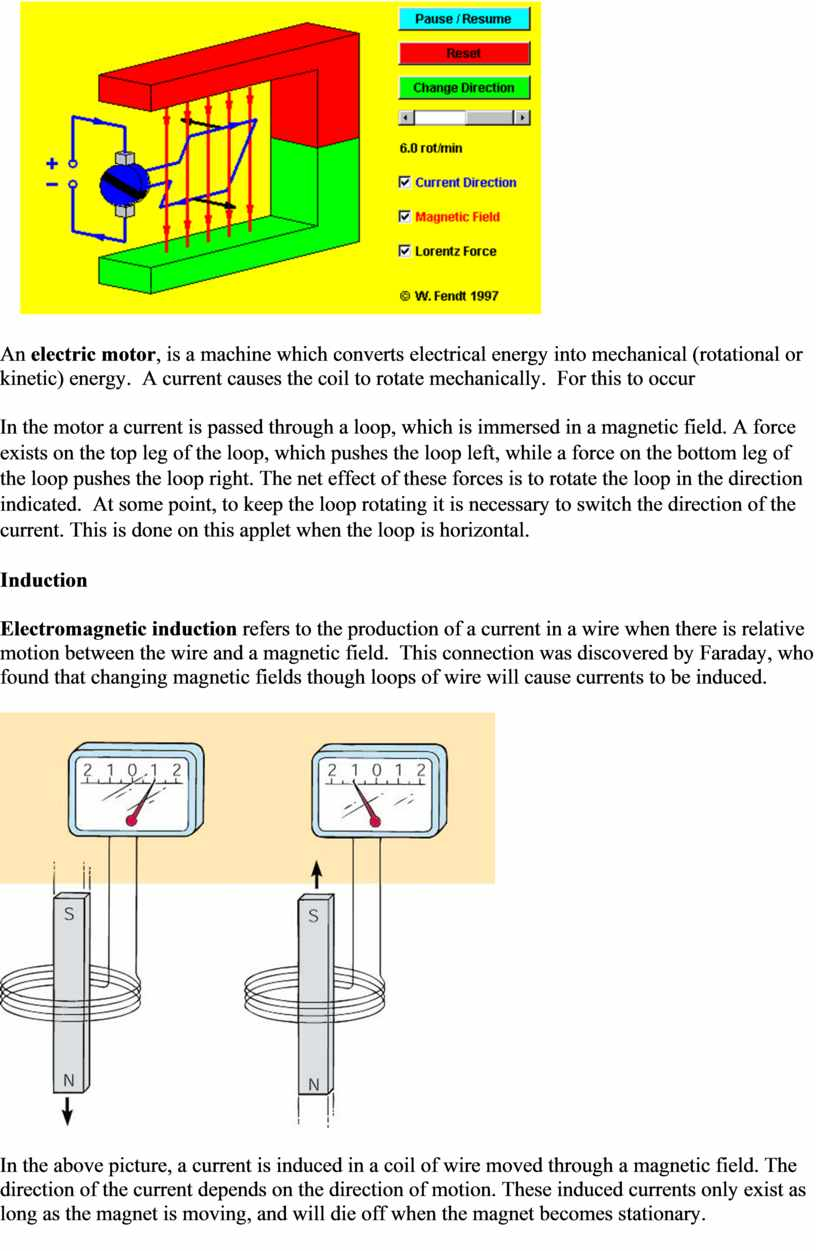## It is interesting to note that the current flows so as to create a magnetic field to oppose the change created by moving the bar…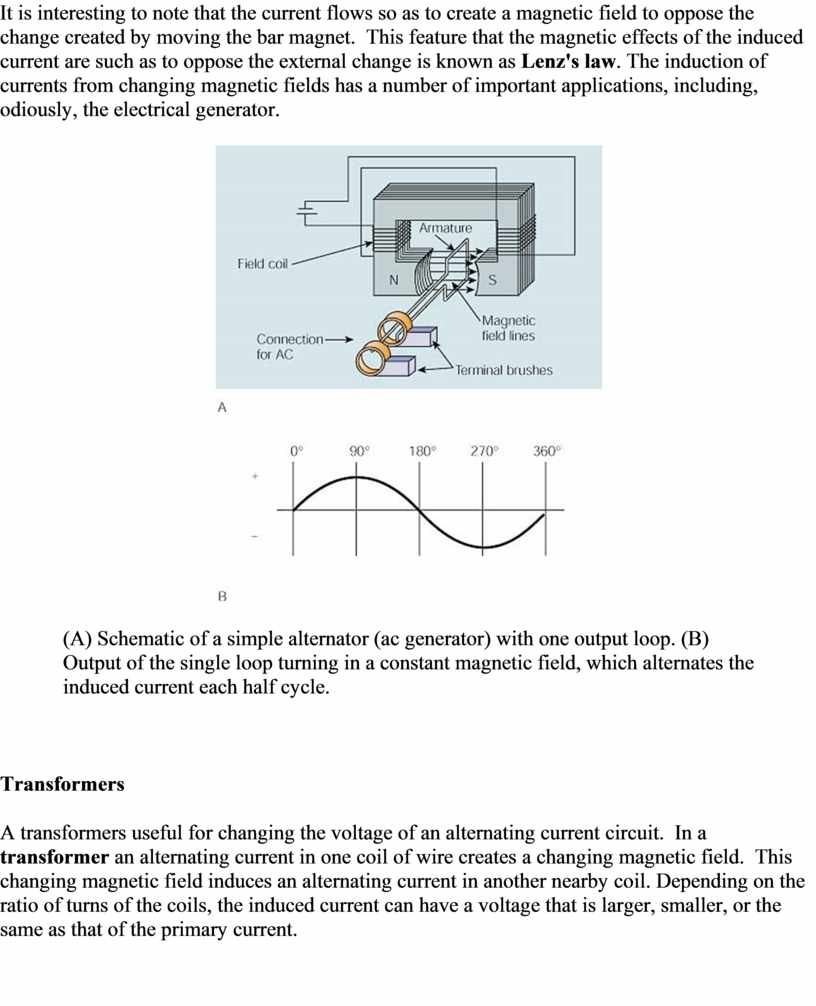## A) This step-down transformer has 10 turns on the primary for each turn on the secondary and reduces the voltage from 120